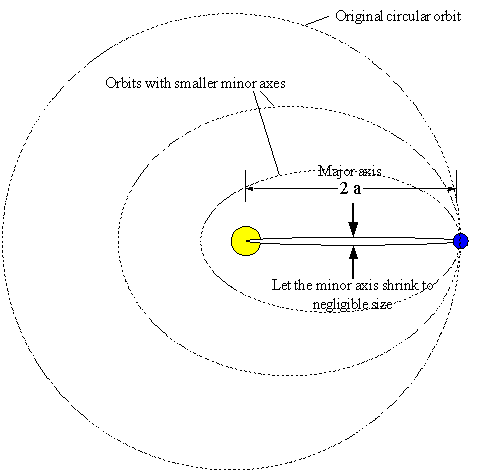# Earth stops moving how long before it hits the sun?

blue.flake

## Homework Statement

So, if the Earth stopped moving(supposing Earth's orbit is a circle) how much time would it have to pass before it hit the Sun?

## Homework Equations

I've tried with Kepler, and Newton's derivations of Kepler's 3th law

## The Attempt at a Solution

Well I'm pretty sure you need to get 64.56 days, but I'm having a few difficulties with the process of getting there.

Also, I'm in a bit of a rush. So if anyone can help I'll be forever in your debt.

Mentor
Hint:

You know Newton's formula for the period of a body in an elliptical orbit? What parameter(s) of the orbit does that formula depend upon?

blue.flake
Well my biggest problem is I get to a point where I have T= √4Π ⋅ (r/2)3 / G ⋅ Ms

and then it seems I should divide T with two, which I don't understand why. Also in this step i don't understand why it's r/2 and not r

Gold Member
what

Have you tried N2L?

If the Earth stops then (classically) it's at rest. Why are you using orbital periods? Maybe I"m misunderstanding the question.

Mentor
Write out your math from the start. What's the basic formula for the period? (You can use μ = G*Msun).

What orbit parameter goes into the period formula? Hint: for circular orbits it happens to be the same as the orbit radius.

You want half the period because when you "drop" the Earth into the Sun you're only interested in the time for it to reach the Sun, not the time for it to reach the Sun and return to its starting place, which would be a full period of the degenerate orbit.

blue.flake
Well honestly I'm completely lost, and at this point totally desperate. I'm really not sure what to do. This is how I done it, but I'm not even sure it's the right way to go

#### Attachments

Mentor
what

Have you tried N2L?

If the Earth stops then (classically) it's at rest. Why are you using orbital periods? Maybe I"m misunderstanding the question.
Integrating an acceleration that depends upon distance in order to solve for time is much more work than using orbit equations in a creative way :)

blue.flake
Is my way the right creative way? :D

Mentor
Newton's version of Kepler's 3rd for elliptical orbits is:
$$T = \frac{2 \pi}{\sqrt{\mu}} a^{\left(3/2\right)}$$
where a is the length of the semi-major axis of the ellipse. Note that the semi-minor axis size is not involved, and that for a circular orbit a is the same as the orbit radius.

Sketch your "degenerate" orbit that's equivalent to the Earth plunging straight into the Sun. Effectively the semi-minor axis shrinks down to negligible width, while the perihelion distance becomes indistinguishable from the Sun's center.

Be sure to indicate the semi-major axis on your diagram!

blue.flake
so a =r? but then I did it right or?

Mentor
so a =r? but then I did it right or?

Mentor
Okay, that's incomplete as you say. What you want to do is consider an elliptical orbit where the temporarily "stationary" Earth is at the aphelion point. As the minor axis of the orbit is shrunk down to negligible size, the perihelion distance pulls closer to the Sun's center.Note that the major axis of the orbit is 2a, where a is called the semi-major axis (half the major axis). For your "degenerate" orbit the whole major axis is the same size as the original orbit radius of the circular orbit.

So, what is the value of "a" for your problem?

blue.flake
ooooh it's r/2 or 1,496*10^11 m divided by 2

Mentor
ooooh it's r/2 or 1,496*10^11 m divided by 2
Right. So that explains why you need to use r/2 in the period formula. Can you also see why you want half the period of the resulting orbit?

blue.flake
Yes because it only falls to the Sun, it doesn't go back. :D

Mentor
Yes because it only falls to the Sun, it doesn't go back. :D

CorrectGold Member
That's pretty clever gneill, and also not how I would have approached that at all. =D